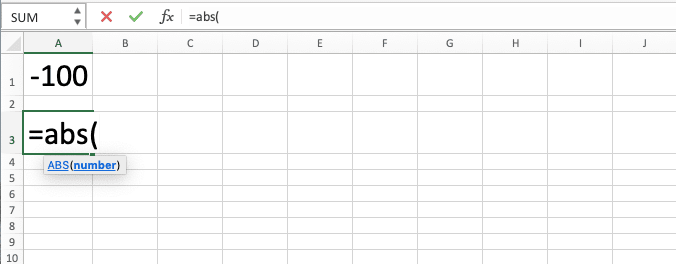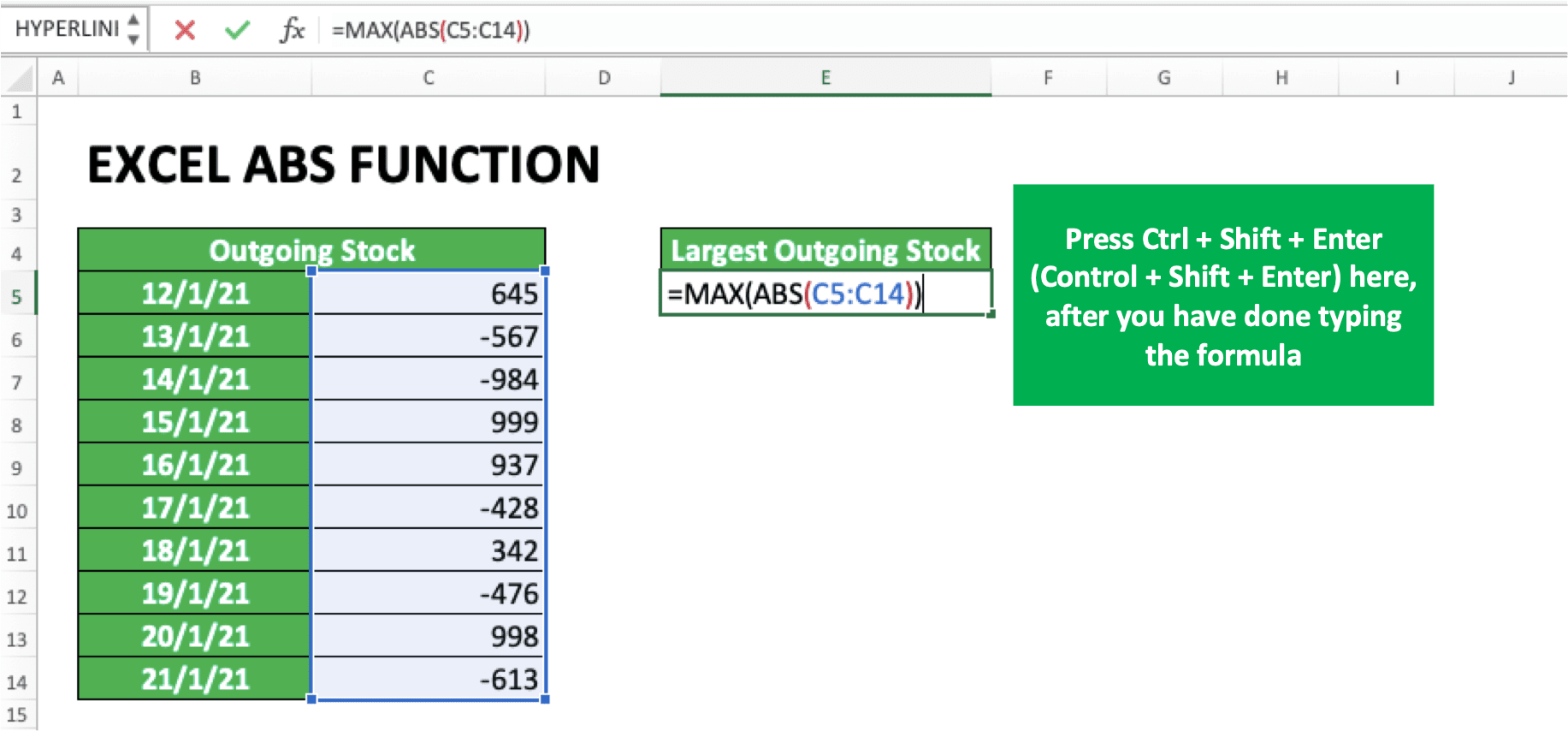How to Use Excel ABS Function: Usability, Examples, and Formula Writing - Compute Expert

# How to Use Excel ABS Function: Usability, Examples, and Formula Writing

Home >> Excel Tutorials from Compute Expert >> Excel Formulas List >> How to Use Excel ABS Function: Usability, Examples, and Formula Writing

In this tutorial, you will learn completely the use of the ABS function in excel. ABS can help to convert the number we input into its absolute form. Excel ABS function is often used with various calculation formulas that need positive numbers, either in its reference or its result.

Disclaimer: This post may contain affiliate links from which we earn commission from qualifying purchases/actions at no additional cost for you. Learn more

## ABS Usefulness

The ABS formula in excel is useful to convert the number which becomes its input into its positive form.

## ABS Result

The result you get from ABS is the positive form of the number which you input in it.

## Excel Version in Which ABS Can Start to Be Used

ABS can start to be used since excel 2003.

## The Way to Write It and Its Input

Here is the example of the ABS writing generally:

=ABS(number)

The only input it needs is a number, which is the number you want to convert into its positive form.

## Example of Usage and Result

The following will give and explain the example of using ABS and its result.In the example, we can see the benefit of using this formula to convert a number into its absolute/positive form. If the number is already absolute, then the formula won’t change anything.

The way to use ABS is also easy because it only needs the input of the number you want to convert. So, don’t be afraid to use it if you need its usability in your numeric data processing!

## Writing Steps

The following will explain how to write an ABS step-by-step. As explained before, ABS is pretty easy to use and only needs the input of a number that you want to make positive.

1. Type an equal sign ( = ) in the cell where you want to put the positive form of your number2. Type ABS (can be with large and small letters) and an open bracket sign after =3. Input the number you want to make a positive or the cell coordinate where the number is4. Type a close bracket sign5. Press Enter
6. The process is done!## ABS Combination With Other Formulas

The ABS formula is usually used with other excel formulas. It will help convert the number result or reference from another formula into its positive form.

How to do this ABS combination with other formulas?

The method is pretty easy. Like other formula combinations in excel, you just need to input ABS in your formula or input your formula into ABS.

As an example of ABS as another formula’s input, let’s say we want to compare a spending number with its limit.As you can see, the spending number in the screenshot above is in its negative form. If we don’t make it positive, then the comparison we want to do with IF will produce a wrong result.

Because negative numbers are always less than positive numbers, however large the spending is, it will be less than the limit. This is as you can see in the screenshot below.By applying ABS to the spending number before it’s compared to its limit, the comparison process can be done correctly. The way to combine IF with ABS is by inputting the spending number into ABS before it is compared. The example is as seen in the following.By using ABS in the IF, we can get our results correctly!

The same method can also be done if you want to make the ABS result as the input of other formulas. Give ABS to the number you want to make positive in the formula so you can get the result you need.

Now, what if you want to make positive the result of a formula using ABS? The method, of course, is by making the formula as the input of your ABS.

The example of this can be seen in the screenshot below.In the example, we want to make positive the SUM result of the spending numbers. This can be done by inputting our SUM writing into ABS.

Do that and the result of SUM or other formulas inputted in ABS will certainly be an absolute/positive number!

## The Use of ABS for All Numbers in a Cell Range Reference in a Formula

The combination method of ABS and other formulas above can be done if the input is just one number or cell. But, what if we want to make all numbers in a cell range reference of a formula positive with ABS? For that, we need the help of the array formula.

The way to write an array formula of ABS approximately looks like this.

{=…ABS(cell_range_that_contains_the_numbers)…}

The three dots (…) in the writing above represents other formula writing which is combined with ABS. Notice the curly bracket around it? That is the symbol of an array formula in excel.

An array formula is used when we input a cell range in a formula that usually uses an individual data input (just like ABS). Note that the curly bracket there cannot be typed directly when we write our formulas.

If so, then how we create an array formula in a cell? We create it by pressing the Ctrl + Shift + Enter (Control + Shift + Enter in Mac) buttons after we have typed our formulas (not the Enter button like usual). Do it and the curly bracket will be automatically added, a sign that our formulas have become array formulas.If we don’t use an array formula, then the MAX ABS in the example above will produce an error/wrong result.Thus, if you need ABS in a formula which input is a cell range, don’t forget to use this array formula!

## Exercise

After you have learned how to use ABS, you can practice your understanding by doing this exercise below!

### Questions

1. Row 1: Convert the figure in column 1 into its absolute form!
2. Row 2: What is the result of the number in column 1 divided by the column 2’s number? Convert all the numbers that are being calculated to positive!
3. Row 3: What is the result of the sum for numbers in columns 1 and 2 divided by the sum the figure in column 3? Convert all the numbers that are being calculated to positive!

If you input non-digit data into ABS, then the result will be a #VALUE error.

Related tutorials you should learn:

Get updated excel info from Compute Expert by registering your email. It's free!